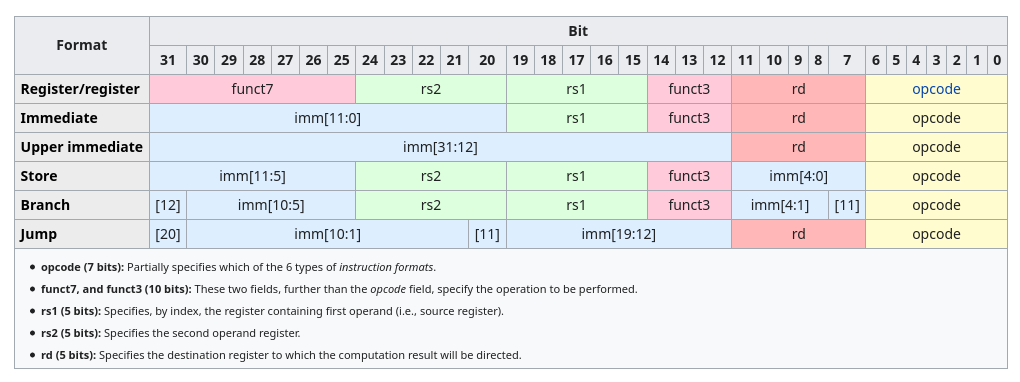Shreyas’ Notes

# ELEC 220

Combine CS and EE to design and implement an optimized computing system.

• the processor gets instructions and data from the memory
• input writes data to the memory
• output reads data from the memory
• control sends the signal that determind the operations of the…
• datapath, which does computations

$\textrm{control} + \textrm{datapath} = \textrm{processor}$

## History §

### Babbage §

• diff engine
• George Scheutz
• analytical engine
• Scheutz

## Binary §

### Unsigned binary integers §

$x = x_{n - 1} 2^{n - 1} + x_{n - 2} 2^{n - 2} + \cdots + x_0 2^0$

Range: $0$ to $2^n - 1$

### 2’s complement signed integers §

$x = -x_{n - 1}2^{n - 1} + x_{n-2} 2^{n-2} + \cdots + x_0 2^0$

MSB is the sign bit.

Range: $-2^{n-1}$ to $+2^{n-1} - 1$

• $0 = {0000 \cdots 0000}_2$
• $-1 = {1111 \cdots 1111}_2$
• Most positive: ${0111 \cdots 1111}_2$
• Most negative: ${1000 \cdots 0000}_2$

### Overflow §

Sum requires more bits than the input data width.

$L - R$ $(+)$ $(-)$
$(+)$ No Maybe
$(-)$ Maybe No
$L + R$ $(+)$ $(-)$
$(+)$ Maybe No
$(-)$ No Maybe

### Sign extension §

move 2’s complement signed int from smaller to larger container: replicate the sign bit to the left

### Floating Points §

• normalized
• not normalized

IEEE 754-1985 standard.

Types:

• single precision — 32 bits
• double precision — 64 bits

Components:

• sign (1 bit)

• exponent (8 vs 11 bits)

implicit bias 😏 (127 vs 1023)

• fraction (23 vs 52 bits)

$x = (-1)^{\mathrm{sign}} \times (1 + \mathrm{fraction}) \times 2^{\mathrm{exponent} - \mathrm{bias}}$

special cases

• $\mathrm{exponent} = 0 \cdots 0$

$x = (-1)^\mathrm{sign} \times (0 + \mathrm{fraction}) \times 2^{-\textrm{bias}}$

• $\mathrm{fraction} \neq 0\cdots 0$

subnormal/denormal numbers.

• $\mathrm{fraction} = 0 \cdots 0$

literally $\pm 0$

• $\mathrm{exponent} = 1 \cdots 1$

• $\mathrm{fraction} \neq 0 \cdots 0$

NaN aka Not a Number.

• $\mathrm{fraction} = 0 \cdots 0$

$\pm \infty$

## Memory §

### Byte ordering §

• Big Endian — LSB has highest address
• Little Endian — LSB has lowest address

Example (0x07AB9DCE):

0x70 07 CE
0x71 AB 9D
0x72 9D AB
0x73 CE 07

• $2^{10}$: Kibibyte (KiB)

• $2^{20}$: Mebibyte (Mib)

• Microprocessor

CPU components. No memory, no I/O.

• Microcomputer

Has memory, some I/O.

• Microcontroller

Many types of memory, sophisticated I/O.

## TM4C123GH6PM §

Memory:

• Flash 256 KiB
• SRAM 32 KiB
• ROM

## C §

### Pointers §

components:

• type: the type of the data at the stored address
1. read operands only from the register file
2. write operations only to the register file
3. only one way to move data between the register file and data memory

### Bitwise operators §

• ~a — not

• a & b — and

x & 0 = 0; x & 1 = x. clear

• a | b — or

x | 0 = x; x | 1 = 1. set

• a ^ b — xor

x ^ 0 = x; x ^ 1 = ~x

• a >> n — right shift

• a << n — left shift

## RISC V §

Reduced Instruction Set Computing.

RISC-V has 32 registers. x0 through x31. Have no type.### R-format — register-register arithmetic §

Operation OP Code funct7 funct3
ADD 0110011 0000000 000
SUB 0110011 0100000 000
SLL 0110011 0000000 001
SLT 0110011 0000000 010
SLTU 0110011 0000000 011
XOR 0110011 0000000 100
SRL 0110011 0000000 101
SRA 0110011 0100000 101
OR 0110011 0000000 110
AND 0110011 0000000 111

### Instructions §

add

• lw — load word

lw dest,offset(source)

• sw — store word

sw dest,offset(source)

• lb — load byte

lb dest,offset(source)

• sb — store byte

sb dest,offset(source)

• slli — shift left (logical)

slli dest,source,bits

• srai — shift right (arithmetic)

• beq — branch (if) equal

• bne — branch (if) not equal

• blt — branch (if) less than

• bge — branch (if) less than

• j — jump

## Analog to Digital §

input: continous time signal

output: discrete sequence

Demultiplexer

Multiplexer

Canonical forms:

• POS
• SOP

Transistors:

• n
• p

## Cache §

memory hierarchy.

trade-off between speed and price/size. small, fast vs large, slow.

locality:

• temporal locality
• spatial locality

types:

• direct-mapped cache
• set associative cache

$C = S \times E \times B$ data bytes

• $S = 2^s$
• $E = 2^e$. when direct-mapped, $e = 1$
• $B = 2^b$

Word length: $t + s + b$